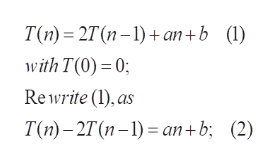Question
9 views

Solve the recurrence relation:

T(n) = 2*T(n-1)+a*n+b

T(0)=0

check_circle

Step 1

To find the formula for T(n), satisfying the given recurrence relation

Step 2

We solve this first by finding the general solution of homogeneous verison (CF) and then the PS (particular solution) of the non-homogeneous parthelp_outlineImage TranscriptioncloseT(n) - 2T (п-1) + an +b (1) with T(0) = 0; Rewrite (1), as T(m) - 2T (п -1) — аn + b; (2) fullscreen
Step 3

clearly (3) sati...

### Want to see the full answer?

See Solution

#### Want to see this answer and more?

Solutions are written by subject experts who are available 24/7. Questions are typically answered within 1 hour.*

See Solution
*Response times may vary by subject and question.
Tagged in

### Math